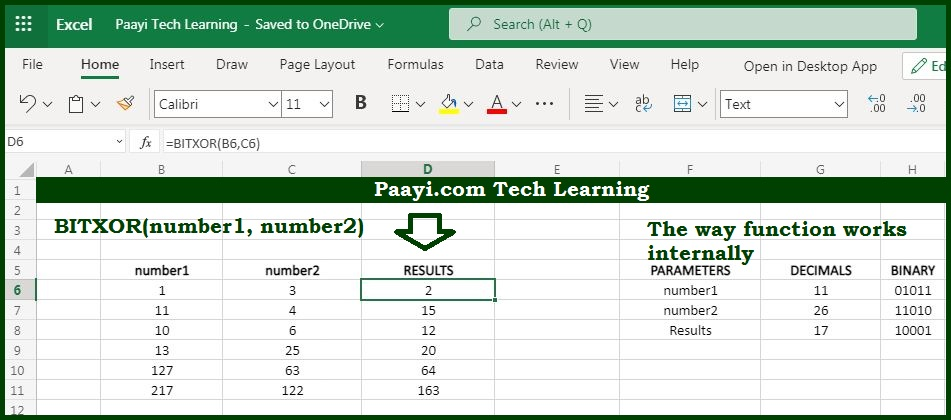# Learn How to Use Microsoft Excel BITXOR Function

Written by | 0 Comments | 737 Views

In this article, you will learn how to use the Microsoft Excel BITXOR function and its prime function in Microsoft Excel. You will also get to know the Microsoft Excel BITXOR function return value and syntax with the help of some examples.

Microsoft Excel BITXOR Function

The main function of the Microsoft Excel BITXOR function is used to return a "Bitwise XOR" of two numbers. That implies with the help of the BITXOR function you can able to return a decimal number that represents the bitwise XOR of the two numbers. For every corresponding bit in the binary representation of the number, the logical XOR operation is performed and the resulting number is returned.

Return Value of BITXOR Function

The return value will be the decimal number.

Syntax of BITXOR Function

=BITXOR(number1, number2)

Where the argument:

• number1: It is the positive decimal number.
• number2: It is the positive decimal number.

## How to Use Microsoft Excel BITXOR Function?It should be kept in mind that the numbers be greater than or equal to zero and must not be longer than 2^48 - 1.

You should understand the basic difference between OR and XOR. Suppose if your mom is asking whether you want tea or coffee, then she is simply asking you can either have tea OR coffee. You won't have both at the same time.